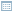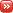• ## Magical Dynamic Python Expressions

Posted by Konstantin 19.06.2010 1 Comment

The other day I was working on a Python program, which internally used a data structure for holding a set of rules. Each rule specified how some object was created from some other objects through certain procedures. The corresponding set of rules could be represented as a bunch of assignment expressions:

`# Description of the relations between objectssome_item.sub_item = some_proc(other_item, "Text", 42)some_item.sub_item = other_proc(some_item, "Text", 1337)# ... etc`

I would manually describe the required structure using code like the following (in fact, it was slightly more complicated than that, but I hope you get the point).

`struct = Structure()struct.add_relation(['some_item', '', '.sub_item'],                  ['some_proc', ['other_item', ''], '"Text"', '42'])struct.add_relation(['some_item', '', '.sub_item'],                 ['other_proc', ['some_item', ''], '"Text"', '1337'])now_do_something_with(struct)`

After having specified the required rules I would process them and thus solve my task, the details of which are not important here. What is important, is that all those `struct.add_relation` lines looked messy, long and ugly, and begged for a more concise syntax.

One obvious choice would be to write the expressions down as strings and parse them within `add_relation` using `ast.parse`:

`struct = Structure()struct.add_relation(  'some_item.sub_item = some_proc(other_item, "Text", 42)')struct.add_relation(  'some_item.sub_item = other_proc(some_item, "Text", 1337)')now_do_something_with(struct)`

Multiple variations on this idea are possible (for example, the whole description could go in a separate configuration file), but in any case this is not a very "easy" solution.

What I am going to show you here is that appropriate use of Python's dynamic features makes it possible to have the structure defined using Python code, side-by-side with normal Python code, in the following manner:

`@structure_definitiondef struct():   some_item.sub_item = some_proc(other_item, "Text", 42)   some_item.sub_item = other_proc(some_item, "Text", 1337) now_do_something_with(struct)`

This post aims to explain the mechanics behind such a solution.

### Part I: __getattr__ and __setattr__

The first step is fairly easy. As you should know, Python is fairly flexible in that it allows you to redefine any operator, including attribute access, function invocation and assignments. We can therefore create a class `StructureCreator`, which does redefine all those operators as needed, and then use it in the following manner:

`s = StructureCreator()s.some_item.sub_item = s.some_proc(s.other_item, "Text", 42)s.some_item.sub_item = s.other_proc(s.some_item, "Text", 1337)`

To see what we need to do to make it work, let us explicitly write out what happens here on line 2. This line is equivalent to the following expression:

`s.__getattr__('some_item') .__getitem__(1) .__setattr__('sub_item',    s.__getattr__('some_proc')    .__call__(s.__getattr__('other_item').__getitem__(2), "Text", 42) )`

This invocation structure neatly corresponds to the parse tree of the original expression and our `StructureCreator` class should therefore simply collect the information from the invocations, recursively building data structures describing the left- and right-hand sides of the expression. Finally, at the time the assignment operator `__setattr__` is invoked, the collected information about the assignment can be saved appropriately. Here is an abridged conceptual example:

`class StructureBuilder:  def __init__(self, expr=''):    self.__dict__['__expr__'] = expr   def __str__(self):    return self.__expr__  __repr__ = __str__   def __getattr__(self, attrname):    newname = self.__expr__ + '.' + attrname    return StructureBuilder(newname)   def __setattr__(self, attrname, val):    newname = self.__expr__ + '.' + attrname    print 'Saving: %s = %s' % (newname, str(val))    s = StructureBuilder()s.x = s.y`

It remains to implement `__getitem__, __setitem__` and `__call__` in a similar manner, and voila, you are all covered in syntax sugar.

The fact that you will have to prefix all your "custom" expressions with this annoying `s.` might still bug your aesthetical feelings. If so, follow me to the next step.

### Part II: Hiding the Builder

In the previous part we figured out how, by using `__getattr__` and `__setattr__` we can make Python interpret assignments our way. In order for it to work, however, we need to explicitly refer to an object which implements those methods, and write lines like

`s.x = s.y`

Can we somehow "hide" this object and have something analogous to automatic `__getattr__` for global scope? Yes we can: Python conveniently provides us with a construction:

`exec <code> in <environment>`

which would execute our code, using the provided dictionary to resolve variables.

Let us try it. We modify our `StructureBuilder` to inherit from `dict` and add the methods `__getitem__` and `__setitem__` which will happily resolve and assign to arbitrary variable names:

`class StructureBuilder(dict):  def __init__(self, expr=''):    self.__dict__['__expr__'] = expr   def __str__(self):    return self.__expr__  __repr__ = __str__   def __getattr__(self, attrname):    newname = self.__expr__ + '.' + attrname    return StructureBuilder(newname)   def __setattr__(self, attrname, val):    newname = self.__expr__ + '.' + attrname    print 'Saving: %s = %s' % (newname, str(val))      def __getitem__(self, itemname):    newname = self.__expr__ + '.' + str(itemname)    return StructureBuilder(newname)   def __setitem__(self, itemname, val):    newname = self.__expr__ + '.' + str(itemname)    print 'Saving: %s = %s' % (newname, str(val)) `

Does it work?

`s = StructureBuilder()exec 'x.y = z' in s`

It does, but now we are providing Python code in a string, which is not at all sexy. Let us try the following instead:

`def definition():  x.y = z exec definition.func_code in s`

Bummer. `NameError: global name 'z' is not defined.`. But why? Isn't that exactly the same as the previous attempt with a string?

### Part III: Lexical Scoping

There is a difference in how Python compiles code in a string and in a function. To see that, let us take a look at what's inside the compiled snippets for a string

`s = 'x = y'`

and a function

`def f():  x = y`

To do that let us use Python's bytecode disassembler module `dis`:

`import dis# First snippet (code in a string)dis.dis(compile(s, '', 'exec'))# Second snippet (code in a function)dis.dis(f.func_code)`

Here is the output for two snippets side by side:

```Snippet1 (String)                Snippet2 (Function)
-------------------------------------------------------
3 STORE_NAME     1 (x)           3 STORE_FAST     0 (x)
9 RETURN_VALUE                   9 RETURN_VALUE ```

Now we see it - the snippet from the string uses the `LOAD_NAME` and `SAVE_NAME` commands to access the variables, which neatly proxy the requests to our dictionary, as we want them to.

The snippet from the function behaves in a different way. Firstly, the variable `x` is accessed using `STORE_FAST`, which is a command used for local variables. This command would not use the provided dictionary because the variable `x` has been determined as local to the function's scope and assigned its own memory location within the function's frame at compilation. Secondly, the variable `y` is accessed via `LOAD_GLOBAL` which, in Python's terms, refers to a variable from a surrounding lexical scope, not the dynamic scope we are trying to enforce. In short, this command also does not care about the dictionary that we provided.

Does it mean that it is impossible to overcome the scoping rules that Python uses when compiling a function? Of course not - all we need is to modify the bytecode, replacing each `LOAD_FAST, STORE_FAST, LOAD_GLOBAL` and `STORE_GLOBAL` with `LOAD_NAME` and `STORE_NAME`.

### Part IV: Bytecode

The required modification is well documented here, but let me give you a brief overview.
The bytecode for a Python function `f` is stored in its field `f.func_code.co_code`. It is a sequence of bytes, which is easy to parse into opcodes. Every opcode is one byte long. Opcodes with a byte-value greater or equal than opcode.HAVE_ARGUMENT are followed by a two-byte argument. This does not seem to be well documented, but is easily read out from the code of the `dis.dis` function, which comes with Python. For completeness' sake, here's how a simple bytecode parser might look like:

`def bytecode(code_obj):	import opcode	code = code_obj.co_code	n = len(code)	i = 0	while i < n:		op = ord(code[i])		i += 1		oparg = None		if op >= opcode.HAVE_ARGUMENT:			oparg = ord(code[i]) + ord(code[i+1])*256			i += 2		yield (op, oparg) `

We now need to take the code object of our function, replace the `***_FAST` and `***_GLOBAL` commands with `***_NAME` and create a new code object. Leaving out some details, this goes as follows:

` def as_anonymous_block(func):  new_bytecode = []  for (op, arg) in bytecode(func.func_code):    if (op in [STORE_FAST, LOAD_FAST, STORE_GLOBAL, LOAD_GLOBAL]):      new_op = LOAD_NAME or STORE_NAME, correspondingly      new_arg = for ***_FAST we need to modify this value      new_bytecode.append(new_op, new_arg)    else:      new_bytecode.append(op, arg)  return types.CodeType( ... options, flags ... , new_bytecode) `

Once we perform this modification on our function object, we can use `exec ... in ... ` to have our `StructureBuilder` take control of the assignments within the function:

`def definition():  x.y = z exec as_anonymous_block(definition) in s `

### Part V: Putting It All Together

As I promised in the beginning of this post, it is possible to encapsulate the whole thing into a function annotation `@structure_definition` and use it as follows:

`@structure_definitiondef struct():   some_item.sub_item = some_proc(other_item, "Text", 42)   some_item.sub_item = other_proc(some_item, "Text", 1337) now_do_something_with(struct)`

The last step is therefore creating the `@structure_definition` annotation, which is rather straightforward. For a given function it processes it using `as_anonymous_block`, creates a new `StructureBuilder` object, `exec`utes the function's code `in` the StructureBuilder, and returns the result:

`def structure_definition(f):  blk = as_anonymous_block(f)  result = StructureBuilder()  exec blk in result  return result `

For further details refer to (a slightly more elaborate) example code here.

To conclude, I should note that in my case I still ended up using the solution from Step I (i.e. the one where "dynamic" variables are prefixed with `s.`), because it allows to use loop variables and other constructs alongside my structure definitions much more naturally than the second method. Nonetheless, I hope you enjoyed the presented exploration as much as I did.

Tags: , , , ,

### Calendar

June 2010
M T W T F S S
« Feb   Mar »
123456
78910111213
14151617181920
21222324252627
282930